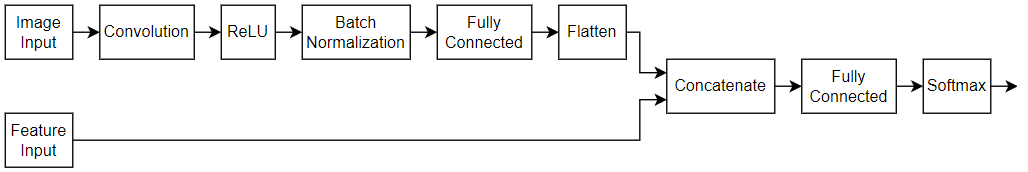# Train Network on Image and Feature Data

This example shows how to train a network that classifies handwritten digits using both image and feature input data.

Load the digits images, labels, and clockwise rotation angles.

`[X1Train,TTrain,X2Train] = digitTrain4DArrayData;`

To train a network with multiple inputs using the `trainNetwork` function, create a single datastore that contains the training predictors and responses. To convert numeric arrays to datastores, use `arrayDatastore`. Then, use the `combine` function to combine them into a single datastore.

```dsX1Train = arrayDatastore(X1Train,IterationDimension=4); dsX2Train = arrayDatastore(X2Train); dsTTrain = arrayDatastore(TTrain); dsTrain = combine(dsX1Train,dsX2Train,dsTTrain);```

Display 20 random training images.

```numObservationsTrain = numel(TTrain); idx = randperm(numObservationsTrain,20); figure tiledlayout("flow"); for i = 1:numel(idx) nexttile imshow(X1Train(:,:,:,idx(i))) title("Angle: " + X2Train(idx(i))) end```### Define Network Architecture

Define the following network.• For the image input, specify an image input layer with size matching the input data.

• For the feature input, specify a feature input layer with size matching the number of input features.

• For the image input branch, specify a convolution, batch normalization, and ReLU layer block with 16 5-by-5 filters.

• To convert the output of the batch normalization layer to a feature vector, include a fully connected layer of size 50.

• To concatenate the output of the first fully connected layer with the feature input, flatten the `"SSCB"`(spatial, spatial, channel, batch) output of the fully connected layer so that it has format `"CB"` using a flatten layer.

• Concatenate the output of the flatten layer with the feature input along the first dimension (the channel dimension).

• For classification output, include a fully connected layer with output size matching the number of classes, followed by a softmax and classification output layer.

Create a layer array containing the main branch of the network and convert it to a layer graph.

```[h,w,numChannels,numObservations] = size(X1Train); numFeatures = 1; numClasses = numel(categories(TTrain)); imageInputSize = [h w numChannels]; filterSize = 5; numFilters = 16; layers = [ imageInputLayer(imageInputSize,Normalization="none") convolution2dLayer(filterSize,numFilters) batchNormalizationLayer reluLayer fullyConnectedLayer(50) flattenLayer concatenationLayer(1,2,Name="cat") fullyConnectedLayer(numClasses) softmaxLayer classificationLayer]; lgraph = layerGraph(layers);```

Add a feature input layer to the layer graph and connect it to the second input of the concatenation layer.

```featInput = featureInputLayer(numFeatures,Name="features"); lgraph = addLayers(lgraph,featInput); lgraph = connectLayers(lgraph,"features","cat/in2");```

Visualize the network in a plot.

```figure plot(lgraph)```### Specify Training Options

Specify the training options.

• Train using the SGDM optimizer.

• Train for 15 epochs.

• Train with a learning rate of 0.01.

• Display the training progress in a plot.

• Suppress the verbose output.

```options = trainingOptions("sgdm", ... MaxEpochs=15, ... InitialLearnRate=0.01, ... Plots="training-progress", ... Verbose=0);```

### Train Network

Train the network using the `trainNetwork` function.

`net = trainNetwork(dsTrain,lgraph,options);`### Test Network

Test the classification accuracy of the network by comparing the predictions on a test set with the true labels.

Load the test data and create a combined datastore containing the images and features.

```[X1Test,TTest,X2Test] = digitTest4DArrayData; dsX1Test = arrayDatastore(X1Test,IterationDimension=4); dsX2Test = arrayDatastore(X2Test); dsTest = combine(dsX1Test,dsX2Test);```

Classify the test data using the `classify` function.

`YTest = classify(net,dsTest);`

Visualize the predictions in a confusion chart.

```figure confusionchart(TTest,YTest)```Evaluate the classification accuracy.

`accuracy = mean(YTest == TTest)`
```accuracy = 0.9834 ```

View some of the images with their predictions.

```idx = randperm(size(X1Test,4),9); figure tiledlayout(3,3) for i = 1:9 nexttile I = X1Test(:,:,:,idx(i)); imshow(I) label = string(YTest(idx(i))); title("Predicted Label: " + label) end```